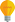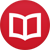# What is $0^0$ ($0$ to the power $0$)?

by Jakub MarianTip: See my list of theMost Common Mistakes in English. It will teach you how to avoid mis­takes with com­mas, pre­pos­i­tions, ir­reg­u­lar verbs, and much more.

A short answer to this question is that $0^0$ is usually left undefined in introductory calculus classes, just like $0/0$, but it is often useful to set $0^0 = 1$ in certain situations. Let’s explain why.

In mathematics, we usually want to extend definitions in a consistent way (in a way that simplifies notation). If it is impossible to keep everything consistent, we are mostly satisfied with leaving the result “undefined”.

The problem with $0^0$ in calculus can be illustrated as follows:

$$1^0 = 1 \\ 0.1^0 = 1 \\ 0.01^0 = 1 \\ … \\ 0^0 = 1\ ?$$

but

$$0^1 = 0 \\ 0^{0.1} = 0 \\ 0^{0.01} = 0 \\ … \\ 0^0 = 0\ ?$$

Mathematically speaking, if you have a function $f(x,y) = x^y$ and you approach $(0,0)$ by varying $x$ and keeping $y = 0$, you get $1$. This can be made precise using the notion of limit:

$$\lim_{x→0} x^0 = 1\,.$$

If you vary $y$ and keep $x = 0$, you get

$$\lim_{y→0} 0^y = 0\,.$$

This proves that the two-dimensional limit at $(0,0)$ does not exist, i.e.

$$\lim_{(x,y) → (0,0)} x^y\ \,\text{does not exist.}$$

At this point, a calculus teacher would proudly proclaim that there is no consistent way to define $0^0$, and he would be right that it makes little sense to define $0^0$ in the context of limits of functions of two variables.

It is reasonable to define, for example, $\frac{1}{∞} = 0$ because $\lim_{x→∞} \frac{1}{x} = 0$, so substituting $\frac{1}{∞}$ for $\lim_{x→∞} \frac{1}{x}$ will simplify notation without increasing the risk of error, but if we defined $0^0 = 1$, it could lead students to believe that any limit of this type is equal to $1$, which is not true.

Nevertheless, there are fields of mathematics where defining $0^0 = 1$ leads to consistent results:

## Combinatorics

Let ${n \choose k}$ denote the binomial coefficient, that is, ${n \choose k} = \frac{n!}{k!(n-k)!}$. One of the most important combinatorial formulas in algebra, which also arises in many other fields of mathematics, is the binomial theorem:

$$(x+y)^n = \sum_{k=0}^n {n \choose k}x^{k}y^{n-k}\,.$$

This formula works for all natural numbers $n$ and real numbers $x$ and $y$, but only if we assume that $0^0 = 1$. For example, for $n = 2$, $x = 0$, and $y = 5$, the formula reduces to

$$(0+5)^2 = 0^0×5^2 + 2×0^1×5^1 + 0^2×5^0\,.$$

If we define $0^0 = 1$, the right-hand side is equal to $5^2$, which is obviously equal to the left-hand side. With this definition, the formula works even for $n=0$, which leads to:

$$(0+5)^0 = 0^0 × 5^0\,.$$

The reason why this works is that, in combinatorics, if you have two sets $M$ and $N$, the number of mappings (functions) from $M$ to $N$ is $|N|^{|M|}$. If both sets are empty (i.e. $|M| = |N| = 0$), there is only one mapping between themthe empty mapping, which does not assign anything to any element.

Whenever you encounter $m^n$ in a combinatorial formula, you can be almost certain that it can be interpreted as the number of mappings between some sets (this is also the case for the binomial formula above, if $x$ and $y$ are natural numbers). As a result, pretty much all combinatorial formulas will work correctly if you define $0^0 = 1$.

## Polynomials

Polynomials (functions like $1+x+x^2$) are used in many different mathematical theories, including calculus. It is often useful to write a polynomial in a compact form as

$$p(x) = ∑_{k=0}^n a_kx^k\,.$$

However, how do you evaluate $p(0)$? If you define $0^0 = 1$, everything will work perfectly. You will get nice formulas for multiplication, derivatives, and all other common operations with polynomials. If you didn’t do that, the polynomial above would have to be written as

$$p(x) = \begin{cases}∑_{k=0}^n a_kx^k & x ≠ 0 \\ a_0 & x = 0\end{cases}\,,$$

which is extremely inconvenient.

## Conclusion

There are good reasons to define $0^0 = 1$ (and I am not aware of any mathematical theory where another definition would be more convenient). The expression is usually left undefined in calculus to remind students that $x^y$ is not a continuous function at $(0,0)$, but setting $0^0 = 1$ would not cause any problems either.

Therefore, defining $0^0 = 1$ seems to be the right decision in virtually any possible context.

By the way, I have written several educational ebooks. If you get a copy, you can learn new things and support this website at the same time—why don’t you check them out?